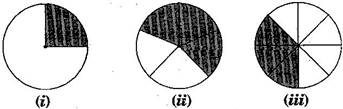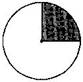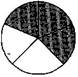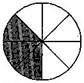### Comparing Quantities - Solutions 2

CBSE Class –VII Mathematics
NCERT Solutions
Chapter 8 Comparing Quantities (Ex. 8.2)

Question 1. Convert the given fractional numbers to percent:
(a) $\frac{1}{8}$
(b) $\frac{5}{4}$
(c) $\frac{3}{40}$
(d) $\frac{2}{7}$
Answer: (a) $\frac{1}{8}$ = $\frac{1}{8}×100\mathrm{%}=\frac{25}{2}\mathrm{%}$ = 12.5%
(b) $\frac{5}{4}$ = $\frac{5}{4}×100\mathrm{%}=$ 5 x 25% = 125%
(c) $\frac{3}{40}$ = $\frac{3}{40}×100\mathrm{%}=\frac{3}{2}×5\mathrm{%}=\frac{15}{2}\mathrm{%}$ = 7.5%
(d) $\frac{2}{7}=\frac{2}{7}×100\mathrm{%}=\frac{200}{7}\mathrm{%}=28\frac{4}{7}\mathrm{%}$
Question 2. Convert the given decimal fractions to percents:
(a) 0.65
(b) 2.1
(c) 0.02
(d) 12.35
Answer: (a) 0.65 = $\frac{65}{100}×100\mathrm{%}$ = 65%
(b) 2.1 = $\frac{21}{10}×100\mathrm{%}$ = 210%
(c) 0.02 = $\frac{2}{100}×100\mathrm{%}$ = 2%
(d) 12.35 = $\frac{1235}{100}×100\mathrm{%}$= 1235%
Question 3. Estimate what part of the figures is coloured and hence find the percent which is coloured:Answer: (i) Coloured part = $\frac{1}{4}$
$\therefore$ Percent of coloured part = $\frac{1}{4}×100\mathrm{%}$ = 25%(ii) Coloured part = $\frac{3}{5}$
$\therefore$ Percent of coloured part = $\frac{3}{5}×100\mathrm{%}$ = 60%(iii) Coloured part = $\frac{3}{8}$
$\therefore$ Percent of coloured part = $\frac{3}{8}×100\mathrm{%}$ = $\frac{3}{2}×25\mathrm{%}$
= 37.5%Question 4. Find:
(a) 15% of 250
(b) 1% of 1 hour
(c) 20% of Rs. 2500
(d) 75% of 1 kg
Answer: (a) 15% of 250 = $\frac{15}{100}×250$ = 15 x 2.5 = 37.5
(b) 1% of 1 hours = 1% of 60 minutes = 1% of (60 x 60) seconds
$\frac{1}{100}×60×60$ = 6 x 6 = 36 seconds
(c) 20% of Rs. 2500 = $\frac{20}{100}×2500$ = 20 x 25 = Rs. 500
(d) 75% of 1 kg = 75% of 1000 g = $\frac{75}{100}×1000$ = 750 g = 0.750 kg
Question 5. Find the whole quantity if:
(a) 5% of it is 600
(b) 12% of it is Rs. 1080
(c) 40% of it is 500 km
(d) 70% of it is 14 minutes
(e) 8% of it is 40 liters
Answer: Let the whole quantity be $x$ in given questions:
(a) 5% of $x$ = 600
$⇒$ $\frac{5}{100}×x=600$
$⇒$ $x=\frac{600×100}{5}$ = 12,000
(b) 12% of $x$ = Rs. 1080
$⇒$$\frac{12}{100}×x=1080$
$⇒$ $x=\frac{1080×100}{12}$ = Rs. 9,000
(c) 40% of $x$ = 500 km
$⇒$ $\frac{40}{100}×x=500$
$⇒$ $x=\frac{500×100}{40}$ = 1,250 km
(d) 70% of $x$ = 14 minutes
$⇒$ $\frac{70}{100}×x=14$
$⇒$ $x=\frac{14×100}{70}$ = 20 minutes
(e) 8% of $x$ = 40 liters
$⇒$ $\frac{8}{100}×x=40$
$⇒$ $x=\frac{40×100}{8}$ = 500 liters
Question 6. Convert given percents to decimal fractions and also to fractions in simplest forms:
(a) 25%
(b) 150%
(c) 20%
(d) 5%
 S. No. Percents Fractions Simplest form Decimal form (a) 25% 25100$\frac{25}{100}$ 14$\frac{1}{4}$ 0.25 (b) 150% 150100$\frac{150}{100}$ 32$\frac{3}{2}$ 1.5 (c) 20% 20100$\frac{20}{100}$ 15$\frac{1}{5}$ 0.2 (d) 5% 5100$\frac{5}{100}$ 120$\frac{1}{20}$ 0.05
Question 7. In a city, 30% are females, 40% are males and remaining are children. What percent are children?
Answer: Given: Percentage of females = 30%
Percentage of males = 40%
Total percentage of females and males = 30 + 40 = 70%
Percentage of children = Total percentage – Percentage of males and females
= 100% – 70%
= 30%
Hence, 30% are children.
Question 8. Out of 15,000 voters in a constituency, 60% voted. Find the percentage of voters who did not vote. Can you now find how many actually did not vote?
Percentage of voted candidates = 60%
Percentage of not voted candidates = 100% – 60% = 40%
Actual candidates, who did not vote = 40% of 15000 = $\frac{40}{100}×15000$ = 6,000
Hence, 6,000 candidates did not vote.
Question 9. Meeta saves Rs. 400 from her salary. If this is 10% of her salary. What is her salary?
Answer: Let Meera’s salary be Rs.$x.$
Now, 10% of salary = Rs. 400
$⇒$ 10% of $x$ = Rs. 400
$⇒$ $\frac{10}{100}×x=400$
$⇒$ $x=\frac{400×100}{10}$
$⇒$ $x=$ 4,000
Hence, Meera’s salary is Rs. 4,000.
Question 10. A local cricket team played 20 matches in one season. It won 25% of them. How many matches did they win?
Answer: Number of matches played by cricket team = 20
Percentage of won matches = 25%
Total matches won by them = 25% of 20 = $\frac{25}{100}×20$ = 5
Hence, they won 5 matches.